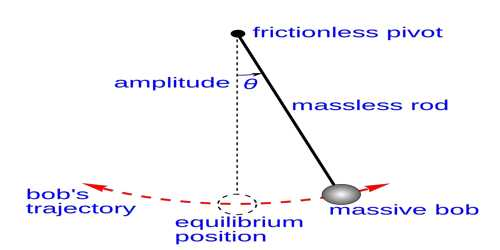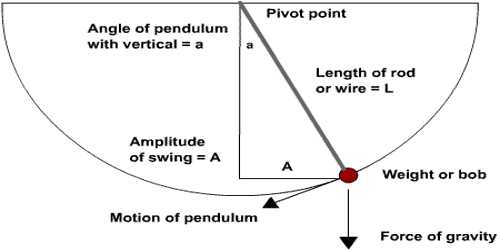Physics

# Third Law of Simple Pendulum – Law of AccelerationThird Law of Simple Pendulum —Law of acceleration: When the angular acceleration does not exceed 4°, the time period of a simple pendulum for a particular length is inversely proportional to square root of the acceleration due to gravity.

If T be the time period and g, the acceleration due to gravity, then T ∞ 1 / √g; if  g increases T will decrease and vice-versa.

If time period at the place of gravitational acceleration g1 and g2 are respectively T1 and T2, then T12 x g1 and T22 x g2.Explanation

The third law of the simple pendulum states that the time period of oscillation of a simple pendulum is directly proportional to the square root of the length of the pendulum, for a given place. That is,

T α √L … … (1)

Equation (1) can be written as

√L/T = constant

On squaring the equation, we get,

L/T2 = constant

Let us now verify the third law of the simple pendulum. Set up the pendulum with a metallic bob as in the experiment to verify the first law. Select a length, say 50 cm. Pull the bob to a side such that the amplitude of the pendulum is not greater than 5 cm and release it. The pendulum starts oscillating. Start the stop watch when the bob is at one of its extreme positions. Count 20 oscillations and stop the stop watch. Note the time taken for 20 oscillations and tabulate the readings under the head trial-one. Repeat the procedure with the same length and amplitude. Note the time taken for 20 oscillations and tabulate the readings under the heads trial-two and trial-three, and so on. Calculate the average time taken for 20 oscillations and determine the time taken for one oscillation. Then tabulate all the readings. Now find the value of L by T square for the given length of the pendulum. Now increase the length of the pendulum by about 10 cm and repeat the procedure to find the value of L/T2. Repeat the procedure three or four times by increasing the length of the pendulum by 10 cm every time. Now tabulate the readings. It will be observed that the time period of the pendulum increases with its length, and the value of L/T2 is the same in all the cases. This verifies the third law.